# How to model a one-way slab in SCIA Engineer?

A one-way slab is a slab that bears the load in one direction mainly. It can be a slab supported on two edges only or a slab supported on four edges for which the bigger span length Ly is at least twice the smaller span Lx. The design of a one-way slab will lead to reinforcement mainly in the bearing direction.

In a Finite Element software like SCIA Engineer, when the slab is supported on its four edges, the software will by default consider it as a two-way slab. Since there is no predefined main direction for the bearing of the load, the bending stiffness of the slab will participate in both x and y directions. In SCIA Engineer the user can easily define a one-way-bearing slab.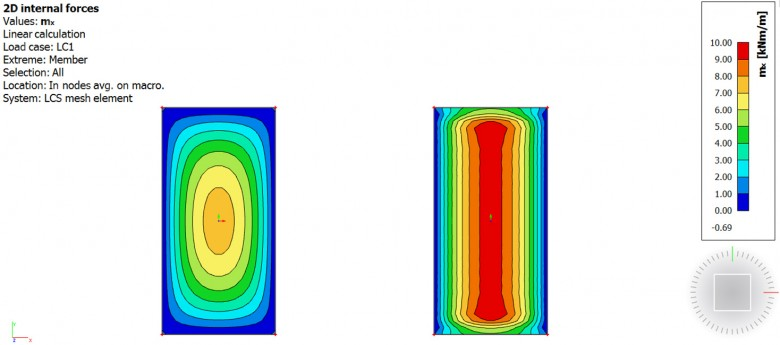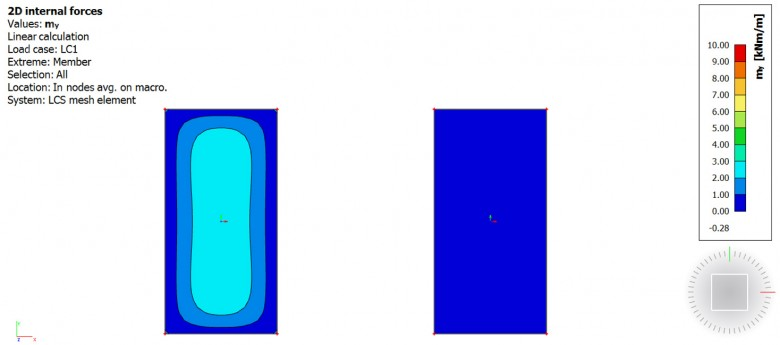Figure 1: Bending moments in a two-way slab (on the left) and one-way slab (on the right)

In SCIA Engineer, the input of a one-way slab can be done with orthotropy properties. Two types can be used and are explained below.

## One-way slab using “two heights” orthotropy type

The example is made of a slab supported by beams and columns. In the slab properties, change the FEM model to “Orthotropy”, edit the orthotropy property and select the type of orthotropy “two heights”. The input data are the thickness of the plate for the calculation of the flexural stiffness in the x-direction, d1, and the y-direction, d2. For a slab bearing mainly in the x direction (smaller span length in the example), d1 should be kept equal to the actual plate thickness (180 mm) and d2 (thickness in y-direction) should be reduced.Figure 2: Parameters for a one-way slab using "two heights" orthotropy type

There is no specific rule regarding the value of d2. With smaller values of d2, the results will be close to the following load distribution: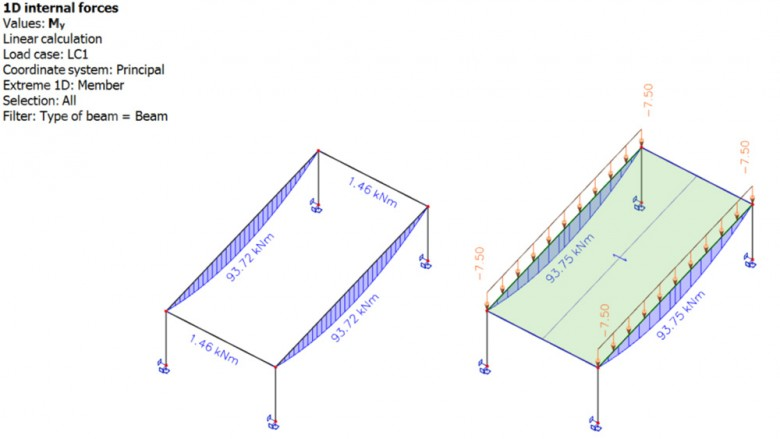Figure 3: Bending moment in the supporting beams of a one-way slab (on the left) and of a one-way load panel (on the right)

The resulting moment mx in the slab is then close to a 1m-wide simply supported beam: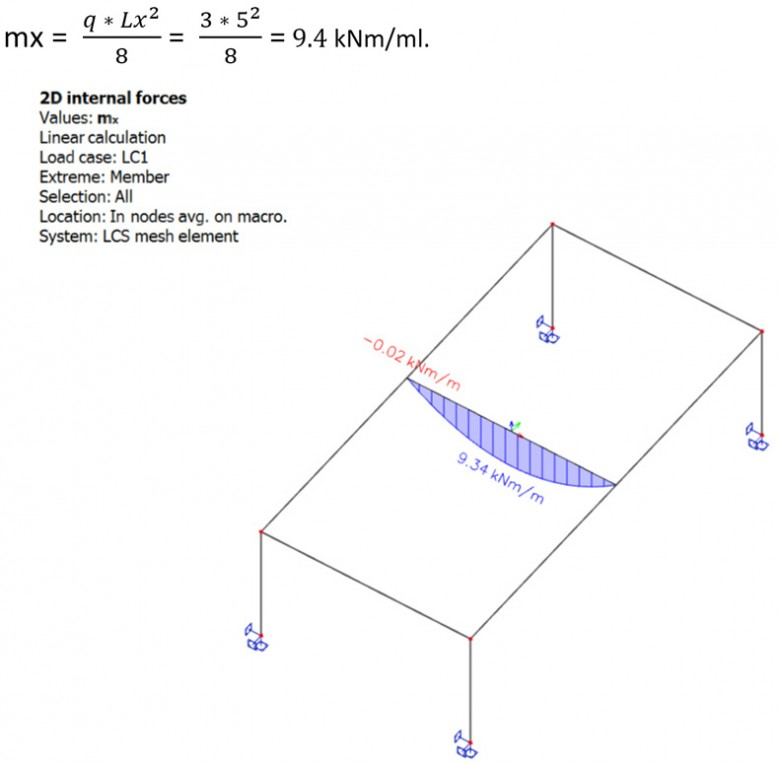Figure 4: bending moment mx in a one-way slab

## One-way slab using “one direction” orthotropy type

This type of orthotropy requires three input parameters and can also be used for modelling hollow core slabs: the equivalent beam cross-section CSS, the spacing L used for the calculation of the flexural bending stiffness in direction 1 (or x) and the concrete topping height h used for the calculation of the flexural bending stiffness in direction 2 (or y). To model a one-way slab, a small value of h can be used. However, keep in mind, that h is also used for the calculation of the self weight of the slab.

For the equivalent cross-section, a slab-equivalent shape is used: “thickness of the slab” x “width of the beam”, i.e. 180 x 1000 mm. For the spacing parameter, as the slab is plain, the same value as for the width of the beam is used, i.e. 1000 mm.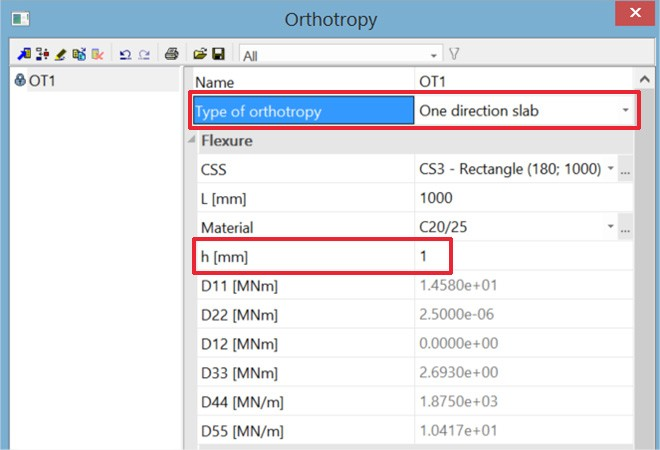Figure 5: Parameters for a one-way slab using "one direction" orthotropy type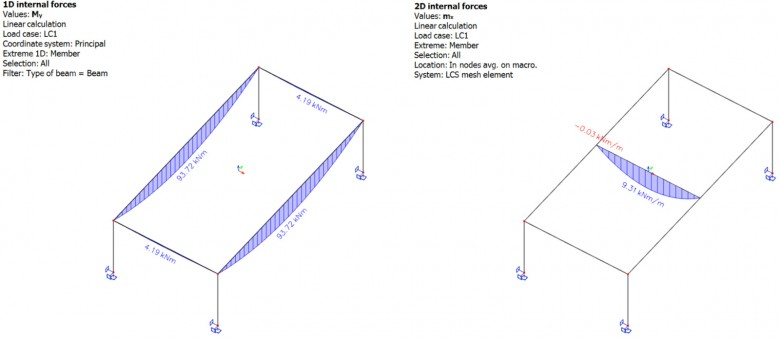Figure 6: Bending moment in the supporting beams and in the one way slab using the type "one direction"

For small values of d2 or h, both types give the same results for the bending moment in the bearing direction and the load transfered to the supported beams.

There are still some differences between both orthotropy types. First, using “one direction” type leads to higher values of bending moment on the secondary beams (parallel to the bearing direction). This is due to the torsional moment component of the plate (D33) that is different between both types. Secondly, with “one direction” orthotropy type, the self weight of the slab is calculated based on the concrete topping thickness h only. The total height of the slab is thus not accounted for and the user has to add the missing part of the self weight manually in a permanent load case.